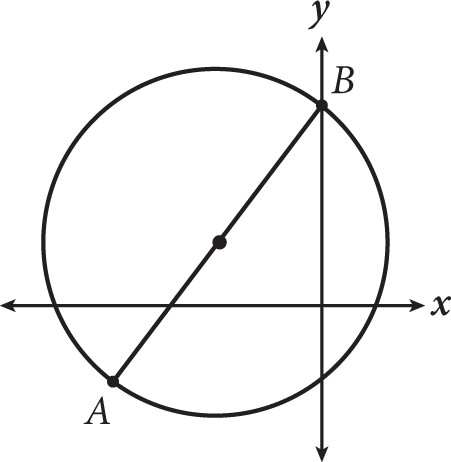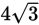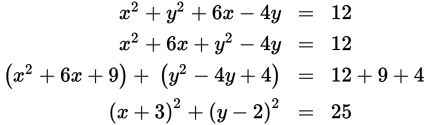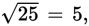# SAT Math Multiple Choice Question 254: Answer and Explanation

### Test Information

Question: 254

14.The circle shown is given by the equation x2 + y2 + 6x - 4y = 12. What is the shortest distance from A to B?

• A. 5
• B. 10
• C.• D. 24

Explanation:

B

Difficulty: Hard

Category: Additional Topics in Math / Geometry

Strategic Advice: Being able to convert equations to different forms is a valuable skill to master. For example, when the equation of a circle is written in the form (x - h)2 + (y - k)2 = r2, you can easily find the center and the radius of the circle.

Getting to the Answer: The shortest distance from A to B is through the center of the circle, along the diameter, which is twice the radius, so you need to find r. To do this, complete the square for the x-terms and for the y-terms. Start by reordering the terms. Then, take the coefficient of the x-term and divide it by 2, square it, and add the result to the two terms with x-variables. Do the same with the y-term. Remember, you must also add these amounts to the other side of the equation. This creates a perfect square of x-terms and y-terms, and the equation will look more like a circle.This means that the radius of the circle isso the diameter is 10, which is also the distance from A to B. Note that you can do a quick check of your work by looking at the center; according to the equation, the center is (-3, 2), which appears to match the location of the center on the graph.